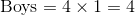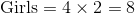ISEE Lower Level Math : Ratio and Proportion

Example Questions

Example Question #11 : Ratio And Proportion

A car with a tank that holds 15 gallons of gas gets 29 miles to the gallon. If the car's gas gauge reads that the tank is three-fourths full, how many miles can it travel before it needs to be refueled?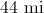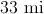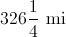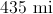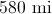Explanation:

Multiply 15 gallons by 29 miles per gallon to get the distance the car can travel on a full tank: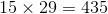miles.

Multiply this by three-fourths to get the distance it can travel on three-fourths of a tank.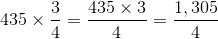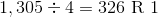, so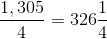miles

Example Question #12 : Ratio And Proportion

There are 24 pencils in a new box.  I have sharpened 18.  What is the ratio of unsharpened pencils to pencils in the new box?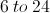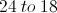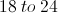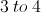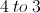Explanation:

The ratio needed is unsharpened to new box and it says there are 24 pencils in a new box.  It does not say how many are unsharpened but it does give the amount that has been sharpened already.

To find the unsharpened amount subtract the number of pencils sharpened from the number of pencils in the new box.  Therefore, it would be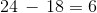unsharpened pencils.

The ratio of unsharpened to new box would be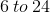.

Example Question #13 : Ratio And Proportion

There are 32 computers in the lab and 30 students from the science class might be using them for a project today.  When it is lab time, 15 students are NOT ready to go.

What is the ratio of students that are NOT ready for lab to students in the science class?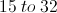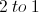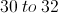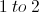Explanation:

The question asked for the ratio of the students NOT ready for lab to the students in the science class.

There are 15 students that are not ready for the computer lab.

There are 30 students in the science class.

Therefore, the ratio is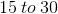.

Since 15 and 30 can be reduced by their greatest common factor of 15 the ratio is simplified to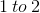.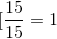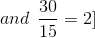Example Question #14 : Ratio And Proportion

A cake recipe asks for 4 cups of flour and 1 cup of sugar. If Sarah only has 2 cups of flour, how much sugar should she use?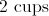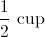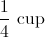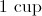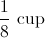Explanation:

In order to solve this problem, we should set up a ratio.

The ratio of flour to sugar in the recipe is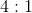If Sarah only has 2 cups of flour, the ratio would be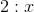whereis the sugar.

Looking at the first ratio, 2 cups is half of 4, so the sugar would also be half. So the answer iscup of sugar.

Example Question #15 : Ratio And Proportion

Mrs. Steven's class has a female-to-male student ratio of 3:2. If the class has 18 female students, how many male students are there?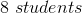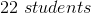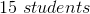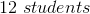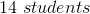Explanation:

The ratio of female-to-male students is 3:2 or:Since we have 18 female students, we can set it up like this: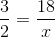whereis the unknown number of male students.

Now multiply the fraction by whatever number so that the numerator (the female students) equals 18.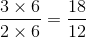So the amount of male students is 12.

Example Question #16 : Ratio And Proportion

A baseball team has a 2:1 ratio of seniors to freshmen, respectively. If there are 27 total players, how many are seniors?Explanation:

Since the ratio of seniors-to-freshmen is 2:1, there are twice as many seniors as freshmen. There's a few ways to solve this. One way is to make the algebra equation: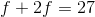Whereis the number of freshmen, and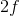is the number of seniors, twice as many as the freshmen.

Now solve for: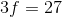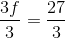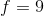But don't forget!is the number of freshmen, and the question asks for seniors, which is: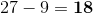Example Question #17 : Ratio And Proportion

There are 7 green chips, 4 black chips, and 8 white chips on a table. What is the ratio of green chips to black chips?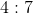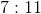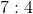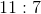Explanation:

The ratio is written as: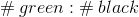or.

The colon (:) indicates the word "to".

Example Question #18 : Ratio And Proportion

A drawer contains 5 pairs of black socks and 7 pairs of red socks. What is the red to black sock ratio?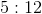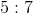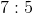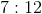Explanation:

A ratio is determined by comparing the number of one item (red socks) to the number of another item (black socks). Therefore, the red to black ratio is.

Example Question #19 : Ratio And Proportion

Michael's math class has 27 students in it.

There are 9 boys and 18 girls.

What is the ratio of boys to girls in Michael's math class?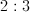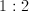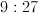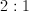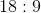Explanation:

There are 9 boys and 18 girls.

This is a ratio of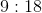Reduce to get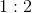Example Question #20 : Ratio And Proportion

The ratio of boys to girls in a group of 12 students is 1 boy to 2 girls.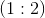. How many girls are in the group?Explanation:

If there is 1 boy for every 2 girls, and there are 12 students, then that means there must be 4 boys and 8 girls.

The ratio ofmeans that there would be 3 students in the smallest group possible (a group with 1 boy and 2 girls).

Thus, if the group has 12 students, we know the group is 4 times bigger than the smallest group possible.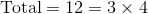This means that we multiply 4 by the ratio to get the number of boys and girls.